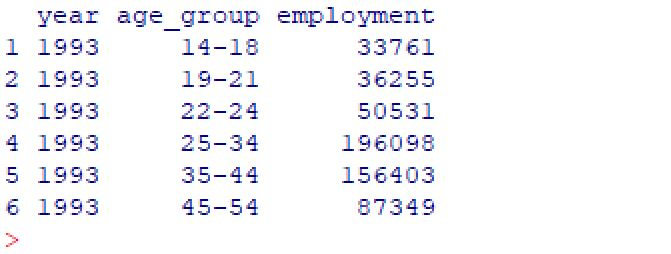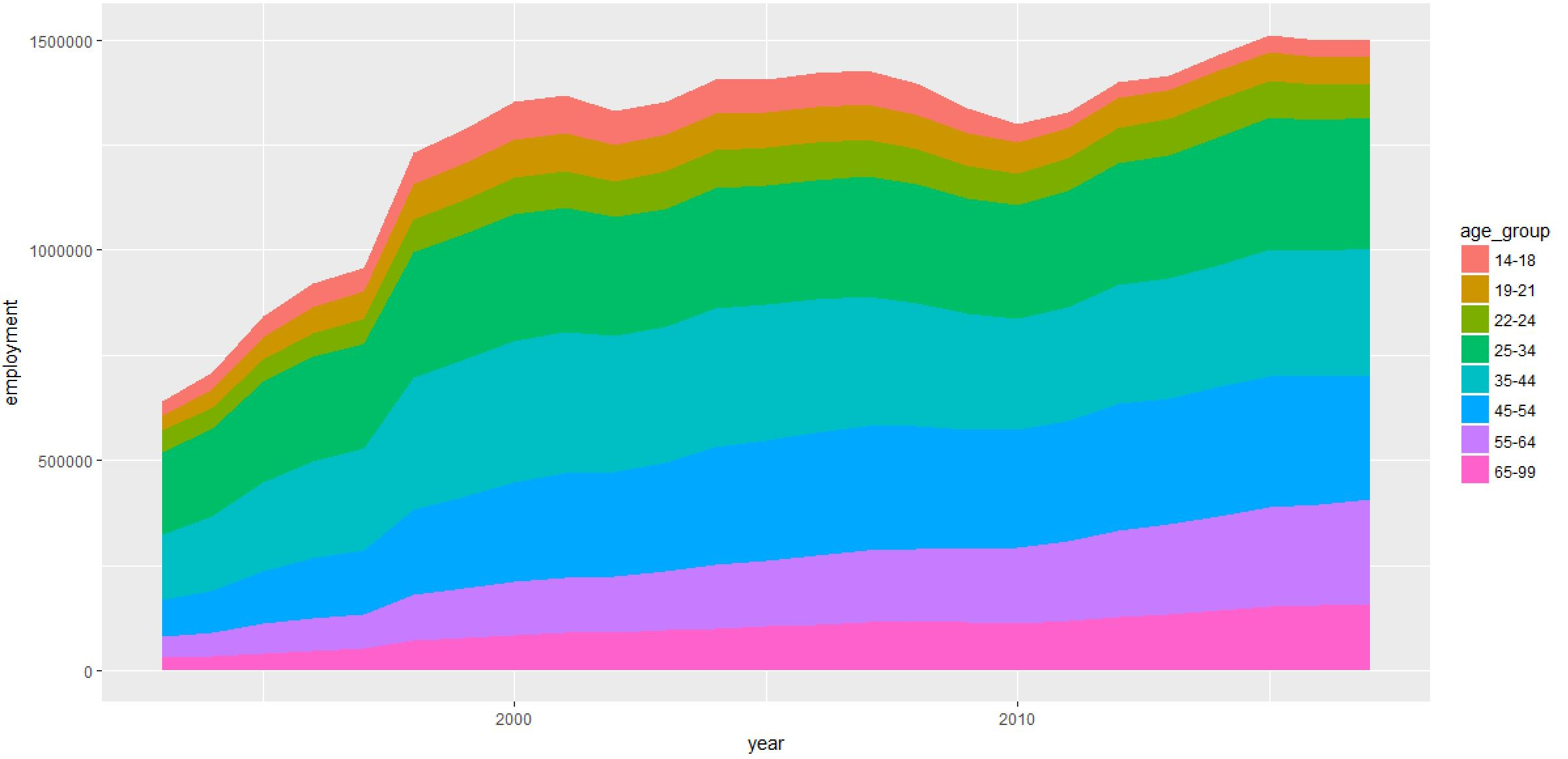Area charts are useful for visualizing one or more variables over time. We can create area charts in R using one of the many available plotting packages. In this article, we'll examine how to create an area chart in R using the ggplot2 package.

Don't forget though, you can easily create an area chart for free using Displayr's free area chart maker!

Before I can make a chart, I need to have some data. For this example, I will load a CSV data set which contains the total number of agriculture jobs by age group in the US from 1993 to 2017.  I add a data frame containing this data by using the read.csv()function, and then preview the data set by using the head()function.

```df = read.csv("employment.csv", header = TRUE)
```
``

We'll now use this data frame to create the area chart using the ggplot2 package.

## Creating an area chart

First, I'll load the ggplot2 library in R.

```library(ggplot2)
```

Next, I call the ggplot() function to create the plot object.

```plot = ggplot(df, aes(x=year, y=employment, fill=age_group))
```

This specifies the data frame to be input to the ggplot function, and it defines which variables will be used as the x-axis, y-axis and fill values. In this case, I want the plot the year on the x-axis with the total employment count on the y-axis. The fill parameter is optional, and if it is omitted a single value area chart will be created. Here I have instead specified the age_group variable, and this will result in a stacked area chart by age group.

Next, I call the geom_area() function to draw the area chart.

```plot + geom_area()
```

``

The above chart was created with the default ggplot2 settings, but there are several chart formatting options that can be modified.  In ggplot2 you can build a plot in stages. This means that you can sequence the functions for modifying the plot by "adding" them with a "+" sign. This is used to separate the different function calls.  The code below applies a series of additional formatting functions to the chart above. I have broken up these functions across multiple lines to help with readability, but these can all be on one line as well.

```plot +
geom_area(colour="black", size=.2, alpha=.8) +
theme_bw() +
scale_x_continuous(breaks=seq(1993,2017,1)) +
scale_y_continuous(breaks=seq(0,2000000,100000)) +
ggtitle("Total US Employment (1993-2017)") +
labs(x="Year", y="Total Employment")
```The code above builds this area chart by:

• Starting with a default area chart.
• Adding standard black/white themed background colors.
• Adding x-axis labels and scalings.
• Adding y-axis labels and scalings.